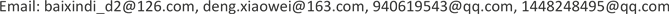1南京工业大学数理科学学院，江苏 南京

2南京工业大学海外教育学院，江苏 南京1. 引言

2018年3月23日特朗普正式签署对华备忘录，宣布将有可能加征关税。自此，本次中美贸易摩擦正式拉开帷幕，之后贸易冲突步步升级。4月16日，美国宣布对中兴通讯的禁购令，导致当天收盘中兴通讯AH股停牌，而美股与之有业务往来的关联股票Acacia Communications Inc.甚至暴跌近36%，资本市场反应逐步传导至A股市场。随后6月、7月美国又逐步签署共2500亿美元的征税清单，项目波及各行各业。资本市场对中美贸易摩擦反应十分敏感，而工业是第二产业的重要组成部分，很大程度上决定着国民经济现代化的速度、规模和水平，在2017年对美贸易前10的上市公司名单中，工业企业就占了6家，作为对美出口贸易的主要行业，同时自然成为了关税清单中的主角，在美国已公布的关税清单细则中，仅化学工业及其相关工业产品就占1442项商品。因此，工业行业股指究竟受到怎样的影响？其在股票市场价格波动中呈现出什么样的变化？这些问题都值得我们研究。

2. 文献综述

3. GARCH族模型

GARCH模型最早由Bollerslev  提出，GARCH模型建立在ARCH模型基础上，将方差方程以自回归移动平均模型的形式描述，变量均取平方形式，使得序列波动对正负冲击做出相同反应。GARCH (p,q)模型的形式如下：

y t = β 0 + ∑ i = 1 p β i y t − i + μ t (1)

σ t 2 = α 0 + ∑ i = 1 p β i σ t − i 2 + ∑ j = a q α j μ t − j 2 (2)

σ t 2 = α 0 + ∑ i = 1 p β i σ t − i 2 + ∑ j = 1 q ( α j + d j α j ) μ t − j 2 (3)

Nelson  将条件方差定义为对数形式，并引入加权扰动函数，提出了EGARCH模型，在反映非对称性效应的同时，去除了参数非负的条件，大大增加了模型的适用性，EGARCH模型的条件方差方程形式如下：

ln ( σ t 2 ) = α 0 + ∑ i = 1 p β i ln ( σ t − i 2 ) + ∑ j = 1 q α j g ( μ t − j σ t − j ) (4)

D = { 0 , 2018 年 3 月 前 ； 1 , 2018 年 3 月 后 .

σ t 2 = α 0 + ∑ i = 1 p β i σ t − i 2 + ∑ j = 1 q ( α j + d j α j ) μ t − j 2 + η D (5)

ln ( σ t 2 ) = α 0 + ∑ i = 1 p β i ln ( σ t − i 2 ) + ∑ j = 1 q α j g ( μ t − j σ t − j ) + η D (6)

4. 实证分析4.1. 数据选取及基本分析

R t = ln S t − ln S t − 1

1) 2018.03.23 特朗普正式签署对华备忘录，宣布将有可能加征关税；

2) 2018.06.15美国政府发布了加征关税的商品清单，至此共公布2500亿美元商品的关税清单；

3) 2018.10.10 中美贸易谈判前夕，美股暴跌。

4.2. 正态性及平稳性检验

Normal test result

R−0.00050.0104−0.91357.3524418.70190.0000

4.3. 建立均值、方差方程

R t = − 0.4066 R t − 4 − 0.7427 R t − 6 + 0.3658 μ t − 4 + 0.6964 μ t − 6 (7)

Parameter comparison of TGARCH model
TGARCH (1,1)TGARCH (2,1)TGARCH (3,1)TGARCH (1,2)TGARCH (2,2)TGARCH (1,3)
AIC值−6.5094−6.5190−6.5122−6.5150−6.5130−6.5107
SC值−6.4265−6.4269−6.4109−6.4229−6.4118−6.4094

Parameter comparison of EGARCH model
EGARCH (1,1)EGARCH (2,1)EGARCH (1,2)EGARCH (2,2)EGARCH (1,3)EGARCH (2,3)
AIC值−6.5223−6.5174−6.5593−6.5586−6.5551−6.5563
SC值−6.4394−6.4253−6.4672−6.4574−6.4538−6.4458

ln ( σ t 2 ) = α 0 + β 1 ln ( σ t − 1 2 ) + α 1 | μ t − 1 σ t − 1 | + α 2 | μ t − 2 σ t − 2 | + γ μ t − 1 σ t − 1 + η D (8)

The regression results of the EGARCH (1,2) mode
α 0α 1α 2β 1γη

t检验P值0.0000***0.0005***0.0000***0.0000***0.0000***0.0025***

4.4. ARCH-LM检验

ARCH-LM test of residua

10.00710.9327−0.00400.9327
20.17070.8431−0.02760.5632
30.92470.42870.07430.1196
40.92780.4476−0.04610.3359
50.82640.53130.03250.4982
60.85130.53090.04750.3263
70.72740.64880.00440.9280

4.5. 实证结果及分析

Analysis of paramete
EGARCH (1,2)模型主要参数
ARCH项系数和0.1411

5. 结论5.1. 总结

5.2. 政策性建议

1) 政府职能部门在贸易摩擦中，采取钉住汇率政策，稳定汇率和广义货币供给，避免多渠道的金融冲击使得高杠杆结构导致企业产出严重下降，并在以上基础上增大企业信贷量，帮助企业渡过难关。

2) 对受中美贸易战影响大的企业，一方面，在股市波动冲击时，增大短期信贷量，维持正常生产；当汇率和广义货币供给等同时受到冲击时，降低资产负债率，避免更大的损失。另一方面，借助国家“一带一路”战略，尽快开拓新的市场，避免单一国家出口带来的国际贸易风险。